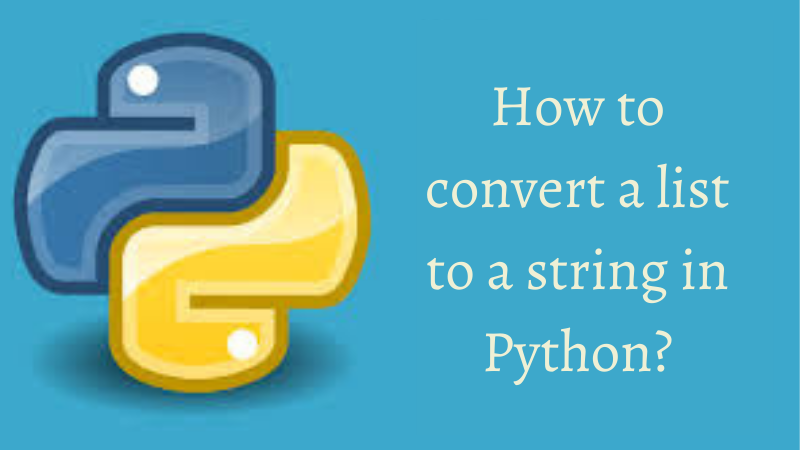/  Technology   /  How to convert a list to a string in Python?## How to convert a list to a string in Python?

We can convert a given list into a string using the below-mentioned ways

• Using manual method
• Using join() method
• Using map() method
• Using list comprehension

Let’s discuss each one of these methods in detail.

#### Using manual method:

We have a list named demo_list with the following elements.

Example:

`demo_list = ['Python ', 'by ', 'i2tutorials']`

We take an empty string named emp_string and using a for loop, we iterate through each element and add that to the empty string. We put the complete code into a function named list_to_string and pass the demo_list as a formal argument.

Example:

```def list_to_string(demo_list):
emp_string = ""
for ele in demo_list:
emp_string += ele
return emp_string

demo_list = ['Python ', 'by ', 'i2tutorials']
print(list_to_string(demo_list))```

Output:#### Using join() function:

The join() method is a string method which returns a string in which the elements of the sequence have been joined by the str separator.

Syntax:

`string_name.join(iterable)`

This method takes an iterable like a List, a Tuple, a String, a Dictionary, a Set, etc. It returns a string concatenated with the elements of the given iterable. This method raises a TypeError exception if the iterable contains any non-string values.

Example:

```def list_to_string(demo_list):
emp_string = ""
return (emp_string.join(demo_list))

demo_list = ['Python ', 'by ', 'i2tutorials']
print(list_to_string(demo_list))```

Output:The above code doesn’t work when the elements of the list are other than the string type. We need to first convert them to string type.

##### Using list Comprehension:

This is a one-liner alternative to the above method. We convert the element first into a string type object after traversing each element of the iterable and add this to the empty string using the join() string method.

Example:

```demo_list = ['Python ', 'by', 'i2tutorials']

list_to_string= ' '.join([str(elem) for elem in demo_list])
print(list_to_string)```

Output:#### Using map():

The map() function returns a map object(which is an iterator) of the results after applying the given function to each item of an iterable like a list, a tuple, etc.

Syntax :

`map(fun, iter)`
• fun is a function to which map() passes each element of the given iterable.
• iter is an iterable which is to be mapped.

This function returns a list with elements after applying the given function to each item of a given iterable like a list, a tuple, etc.

Note: We can pass one or more iterables to the map() function.

Example:

```demo_list = ['Python ', 'by', 'i2tutorials']

list_to_string= ' '.join(map(str, demo_list))
print(list_to_string)```

Output: